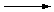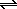Oxidizing and Reducing Agents

Oxidizing and Reducing Agents

Common Oxidizing Agents and Reducing Agents

In looking at oxidation-reduction reactions, we can focus on the role played by a particular reactant in a chemical reaction. What is the role of the permanganate ion in the following reaction, for example?

2 MnO4-(aq) + 5 H2C2O4(aq) + 6 H+(aq)10 CO2(g) + 2 Mn2+(aq) + 8 H2O(l)

Oxalic acid is oxidized to carbon dioxide in this reaction and the permanganate ion is reduced to the Mn2+ ion.

 Oxidation: H2C2O4CO2 +3 +4 Reduction: MnO4-Mn2+ +7 +2

The permanganate ion removes electrons from oxalic acid molecules and thereby oxidizes the oxalic acid. Thus, the MnO4- ion acts as an oxidizing agent in this reaction. Oxalic acid, on the other hand, is a reducing agent in this reaction. By giving up electrons, it reduces the MnO4- ion to Mn2+.

Atoms, ions, and molecules that have an unusually large affinity for electrons tend to be good oxidizing agents. Elemental fluorine, for example, is the strongest common oxidizing agent. F2 is such a good oxidizing agent that metals, quartz, asbestos, and even water burst into flame in its presence. Other good oxidizing agents include O2, O3, and Cl2, which are the elemental forms of the second and third most electronegative elements, respectively.

Another place to look for good oxidizing agents is among compounds with unusually large oxidation states, such as the permanganate (MnO4-), chromate (CrO42-), and dichromate (Cr2O72-) ions, as well as nitric acid (HNO3), perchloric acid (HClO4), and sulfuric acid (H2SO4). These compounds are strong oxidizing agents because elements become more electronegative as the oxidation states of their atoms increase.

Good reducing agents include the active metals, such as sodium, magnesium, aluminum, and zinc, which have relatively small ionization energies and low electro-negativities. Metal hydrides, such as NaH, CaH2, and LiAlH4, which formally contain the H- ion, are also good reducing agents.

Some compounds can act as either oxidizing agents or reducing agents. One example is hydrogen gas, which acts as an oxidizing agent when it combines with metals and as a reducing agent when it reacts with nonmetals.

 2 Na(s) + H2(g)2 NaH(s) H2(g) + Cl2(g)2 HCl(g)

Another example is hydrogen peroxide, in which the oxygen atom is in the -1 oxidation state. Because this oxidation state lies between the extremes of the more common 0 and -2 oxidation states of oxygen, H2O2 can act as either an oxidizing agent or a reducing agent.The Relative Strengths of Oxidizing and Reducing Agents

Spontaneous oxidation-reduction reactions convert the stronger of a pair of oxidizing agents and the stronger of a pair of reducing agents into a weaker oxidizing agent and a weaker reducing agent. The fact that the following reaction occurs, for example, suggests that copper metal is a stronger reducing agent than silver metal and that the Ag+ ion is a stronger oxidizing agent than the Cu2+ ion.

 Cu(s) + 2 Ag+(aq)Cu2+(aq) + 2 Ag(s) stronger reducing agent stronger oxidizing agent weaker oxidizing agent weaker reducing agent

On the basis of many such experiments, the common oxidation-reduction half-reactions have been organized into a table in which the strongest reducing agents are at one end and the strongest oxidizing agents are at the other, as shown in the table below. By convention, all of the half-reactions are written in the direction of reduction. Furthermore, by convention, the strongest reducing agents are usually found at the top of the table.

The Relative Strengths of Common Oxidizing Agents and Reducing Agents

 K+ + e-K Best Ba2+ + 2 e-Ba reducing Ca2+ + 2 e-Ca agents Na+ + e-Na Mg2+ + 2 e-Mg H2 + 2 e-2 H- Al3+ + 3 e-Al Mn2+ + 2 e-Mn Zn2+ + 2 e-Zn Cr3+ + 3 e-Cr S + 2 e-S2- 2 CO2 + 2 H+ + 2 e-H2C2O4 Cr3+ + e-Cr2+ Fe2+ + 2 e-Fe Co2+ + 2 e-Co Ni2+ + 2 e-Ni Sn2+ + 2 e-Sn Pb2+ + 2 e-Pb Fe3+ + 3 e-Fe 2 H+ + 2 e-H2 S4O62- + 2 e-2 S2O32- Sn4+ + 2 e-Sn2+ Cu2+ + e-Cu+ O2 + 2 H2O + 4 e-4 OH- Cu+ + e-Cu I2 + 2 e-2 I- oxidizing MnO4- + 2 H2O + 3 e-MnO2 + 4 OH- power O2 + 2 H+ + 2 e-H2O2 Reducing increases Fe3+ + e-Fe2+ power Hg22+ + 2 e-2 Hg increases Ag+ + e-Ag Hg2+ + 2 e-Hg H2O2 + 2 e-2 OH- HNO3 + 3 H+ + 3 e-NO + 2 H2O Br2(aq) + 2 e-2 Br- 2 IO3- + 12 H+ + 10 e-I2 + 6 H2O CrO42- + 8 H+ + 3 e-Cr3+ + 4 H2O Pt2+ + 2 e-Pt MnO2 + 4 H+ + 2 e-Mn2+ + 2 H2O O2 + 4 H+ + 4 e-2 H2O Cr2O72- + 14 H+ + 6 e-2 Cr3+ + 7 H2O Cl2(g) + 2 e-2 Cl- PbO2 + 4 H+ + 2 e-Pb2+ + 2 H2O MnO4- + 8 H+ + 5 e-Mn2+ + 4 H2O Au+ + e-Au H2O2 + 2 H+ + 2 e-2 H2O Co3+ + e-Co2+ Best S2O82- + 2 e-2 SO42- oxidizing O3(g) + 2 H+ + 2 e-O2(g) + H2O agents F2(g) + 2 H+ + 2 e-2 HF(aq)

Fortunately, you don't have to memorize these conventions. All you have to do is remember that the active metals, such as sodium and potassium, are excellent reducing agents and look for these entries in the table. The strongest reducing agents will be found at the corner of the table where sodium and potassium metal are listed.

 Practice Problem 9:Arrange the following oxidizing and reducing agents in order of increasing strength:           Reducing agents: Cl-, Cu, H2, H-, HF, Pb, and Zn           Oxidizing agents: Cr3+, Cr2O72-, Cu2+, H+, O2, O3, and Na+ Click here to check your answer to Practice Problem 9.

 Practice Problem 10:Predict whether the following oxidation-reduction reactions should occur as written: (a) 2 Ag(s) + S(s)Ag2S(s) (b) 2 Ag(s) + Cu2+(aq)2 Ag+(aq) + Cu(s) (c) MnO4-(aq) + 3 Fe2+(aq) + 2 H2O(l)MnO2(s) + 3 Fe3+(aq) + 4 OH-(aq) (d) MnO4-(aq) + 5 Fe2+(aq)+ 8 H+(aq)Mn2+(aq) + 5 Fe3+(aq) + 4 H2O(l) Click here to check your answer to Practice Problem 10.

 Practice Problem 11:Which of the following pairs of ions cannot exist simultaneously in aqueous solutions? (a) Cu+ and Fe3+     (b) Fe3+ and I-     (c) Al3+ and Co2+ Click here to check your answer to Practice Problem 11.